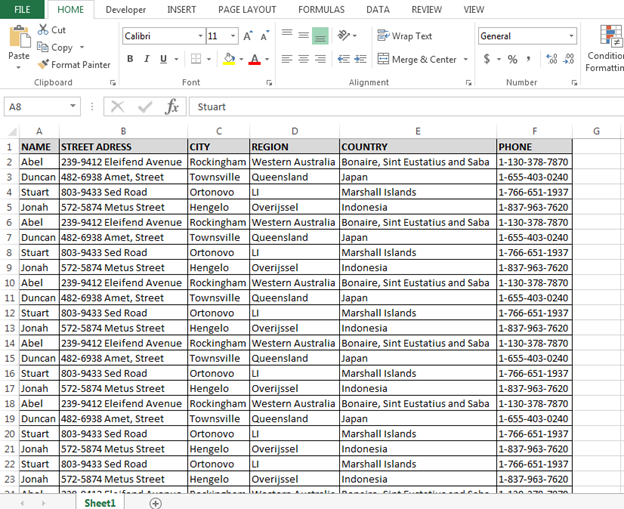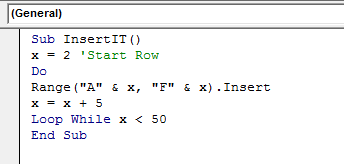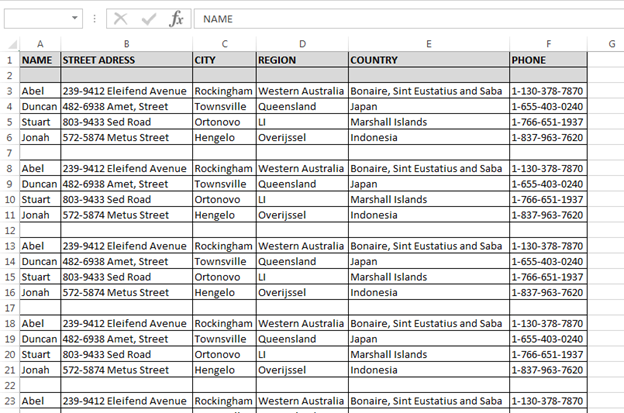How to Insert the Row after Every 5th Row through VBA

In this article, we will learn how to insert the row after every 5th row through VBA in Microsoft Excel.

Let’s take an example and understand:

We have data in range A1:F31, in which column A contains Name, column B contains Street address, column C City, column D contains region, column E contains country name, and column F contains phone number.We want to insert the row after every fifth row in the data, follow below given steps and code:

• Open the VBE page to press the key Alt+F11
• Insert the module to write the code
• Write the below mentioned code:

```Sub InsertIT()

x = 2 'Start Row

Do

Range("A" & x, "F" & x).Insert

x = x + 5

Loop While x < 50

End Sub
```

Code Explanation:

First, we have defined the row number from where we want to insert the row. Then we have defined the range to insert the data. And, then we have defined the number to insert the row after how many rows. And then till which row, rows will be inserted.To run the code press the key F5 on your keyboard.

• Rows will get inserted till the 50th  row in the dataIn this way, we can insert row after specific number which we can define in macro.